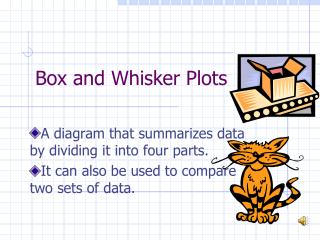DownloadDownload PresentationBox and Whisker Plots

# Box and Whisker Plots

Télécharger la présentation## Box and Whisker Plots

- - - - - - - - - - - - - - - - - - - - - - - - - - - E N D - - - - - - - - - - - - - - - - - - - - - - - - - - -
##### Presentation Transcript

1. Box and Whisker Plots A diagram that summarizes data by dividing it into four parts. It can also be used to compare two sets of data.

2. Box and Whisker Plots The four parts...

3. When plotting on a Box and Whisker plot FIRST • Find median of given data • Find the median of the upper half which is the upper quartile (UQ). • Find the median of the lower half which is the lower quartile (LQ).

4. Second • Graph the median on the number line. • Graph the quartiles on the number line. • Graph the lower and upper extreme.(biggest and smallest value.)

5. Third • Draw a box around the quartiles • Draw a vertical line at the median.

6. Fourth • Draw a line from the quartiles to the extremes

7. Interquartile range Another way to describe how the data are spread out Difference between the upper quartile and the lower quartile. 89 - 75 = 14 = IR Just a note…QUARTILES – can refer to any of the four parts Of a Box-and-Whisker Plot; each quartile contains one-fourth, Or 25%, of the data.

8. Outliers Data that is more than 1.5 times the interquartile range from the quartiles… Example: Upper Quartile =89 Lower Quartile =75 Inter quartile range is 14 (because 89 - 75 = 14) 14*1.5=21 UQ+21= 89+21= 110 LQ-21= 75-21=54 Outliers will be below 54 and above 110. Plot the outlier using a dot.

9. Ben’s Test Scores Describe the data in a sentence or two.